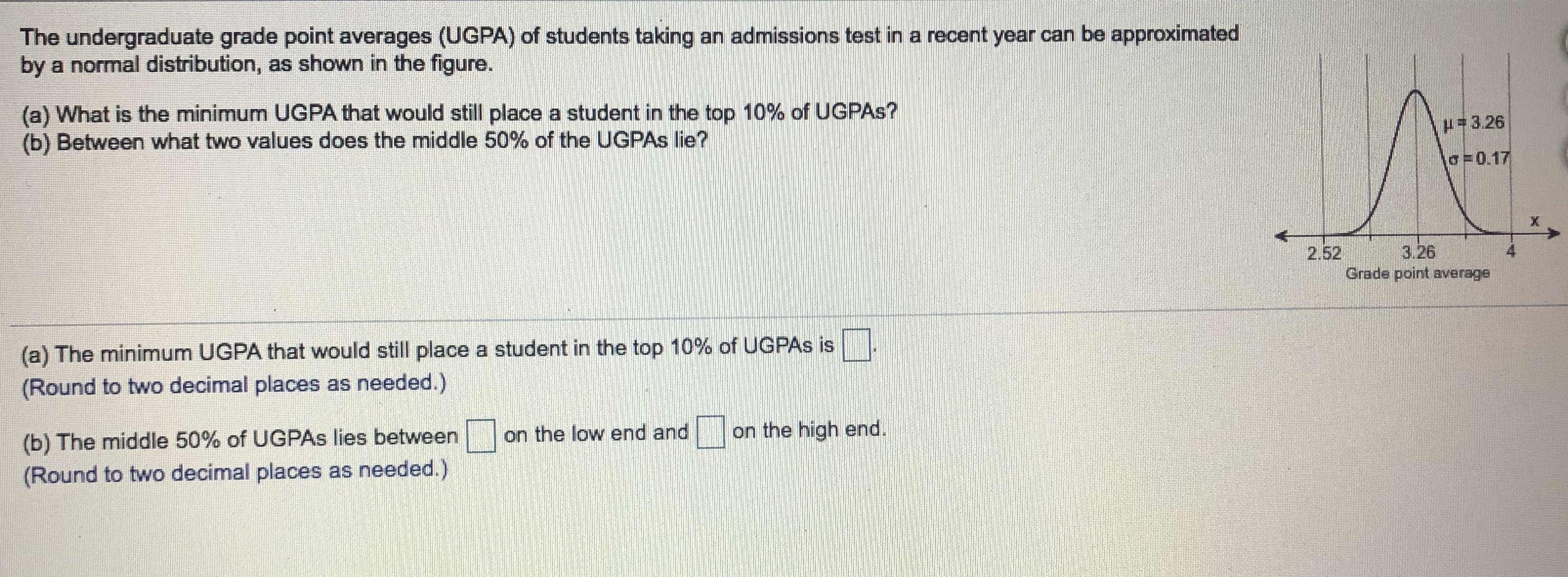# The undergraduate grade point averages (UGPA) of students taking an admissions test in a recent year can be approximatedby a normal distribution, as shown in the figure.(a) What is the minimum UGPA that would still place a student in the top 10% of UGPAS?(b) Between what two values does the middle 50% of the UGPAS Ilie?#3.26o=0.173.262.52Grade point average(a) The minimum UGPA that would still place a student in the top 10% of UGPAS is(Round to two decimal places as needed.)on the high end.on the low end and(b) The middle 50% of UGPAS lies between(Round to two decimal places as needed.)4.

Question
16 viewshelp_outlineImage TranscriptioncloseThe undergraduate grade point averages (UGPA) of students taking an admissions test in a recent year can be approximated by a normal distribution, as shown in the figure. (a) What is the minimum UGPA that would still place a student in the top 10% of UGPAS? (b) Between what two values does the middle 50% of the UGPAS Ilie? #3.26 o=0.17 3.26 2.52 Grade point average (a) The minimum UGPA that would still place a student in the top 10% of UGPAS is (Round to two decimal places as needed.) on the high end. on the low end and (b) The middle 50% of UGPAS lies between (Round to two decimal places as needed.) 4. fullscreen
check_circle

Step 1

(a)

The minimum UGPA that would still place a ...

### Want to see the full answer?

See Solution

#### Want to see this answer and more?

Solutions are written by subject experts who are available 24/7. Questions are typically answered within 1 hour.*

See Solution
*Response times may vary by subject and question.
Tagged in

### Other